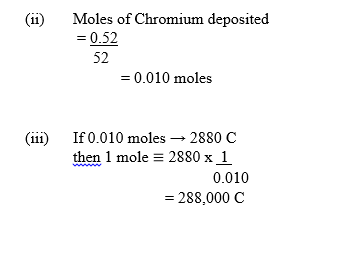Question 4

(a) (i) Name the ore mostly used in the extraction of aluminium.
(ii) Name two major impurities in the ore named in 4(a)(i).
(iii) Name the material used in making the electrodes in the extraction of aluminium.
(iv) Give two reasons why aluminium is commonly recycled.
(v) Explain briefly why the anode has to be replaced at regular intervals during the extraction of aluminium. [8 marks]

(b) A current of 0.75 amperes was passed through an electrolysis containing chromium ions for one hour and four minutes. If the mass of chromium deposited was 0.52 g, calculate the:

(i) quantity of electricity passed;
(ii) moles of chromium deposited;
(iii) quantity of electricity required to deposit one mole of chromium;
(iv) charge on the chromium ion.
[Cr = 52.0, 1 F = 96500 C] [8 marks]

(c) In the contact process for the manufacture of tetraoxosulphate (VI) acid, the following reaction occurs:
2SO2(g) + O2(g) 2SO3 (g) H = - 197 kJ mol-1
(i) Name the catalyst used in the reaction;
(ii) State the optimum temperature for this reaction;
(iii) What would be the effect on the yield of SO3 if a temperature higher than the optimum is used? [3 marks]

(d)(i) State two chemical methods by which temporary hardness of water can be removed.
(ii) Write a balanced chemical equation for each of the methods stated in 4(d)(i).

Observation

In part (a), majority of the candidates could only name the ore of aluminium. However, majority of them could not name two major impurities in the ore and the material used in making the electrodes in the extraction of aluminium.

In part (b), majority of the candidates calculated the quantity of electricity passed and moles of chromium deposited in the electrolysis.

In part (c), majority of the candidates named the catalyst used in the reaction for the manufacturing of tetraoxosuphate (VI) by contact process. However, majority of them could not state the optimum temperature for this reaction.

In part (d), majority of the candidates stated two chemical methods by which temporary hardness of water can be removed. However, majority of them could not write chemical equations for each of the methods.

(a) (i) - Bauxite
(ii) - Iron (III) oxide
- Silica / silicon (IV) oxide
- Calcium trioxosilicate (IV)

(iii)- Graphite / Carbon

(iv) - uses less energy / power / electricity
- conserves resources of ores / bauxite
- less expensive/reduces cost
(v)- Carbon anode reacts with oxygen
- Gas / CO2 given off
(b)(i) Q = It
Q = 0.75 x 64 x 60
= 2880C(iv) Number of Faradays = 288,000
96,500
= 2.984
charge = + 3

(ii) 400 – 500oC / 673 – 773 K
(iii) the yield decreases

(d)(i) - by boiling
- by addition of(calculated amount of) Ca(OH)2 – Calcium hydroxide/slaked lime
- by addition of washing soda/Na2C03
- by addition of NaOH- Sodium hydroxide/Caustic Soda
- by ion exchange/ permutit

(ii)- Ca(HCO3)2(aq) boil CaCO3(s) + H2O(l) + CO2(g)
- Ca(HCO3)2(aq) + Ca(OH)2(aq) → 2CaCO3(s) + 2H2O(l)
- Ca(HCO3)2(aq) + Na2CO3(s) → CaCO3(s) + 2NaHCO3(aq)
- Ca(HCO3)2(aq) + 2NaOH(aq) → 2NaHCO3(aq) + Ca(OH)2(aq)
- Ca(HCO3)2(aq) + 2NaR → CaR2 + 2NaHCO3aq)